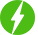# Applications of Smith Chart

0

Applications of Smith Chart, Calculations of transmission line parameters using Smith Chart.

## Course Description

Calculation of transmission line matching circuit parameters using analytical approach is lengthy process, but Smith Chart provides easy solutions to such problems. It is most commonly used tool in microwave and RF engineering to visualize complex quantities.

Smith Chart is circular plot and provides graphical representation of a complex mathematical equations.  It is also used to display multiple parameters simultaneously some of the parameters are impedance, admittance, reflection coefficient, scattering parameters.

Smith Chart consists of two sets of circles. First set is real circles (complete circles) whose center lie on the horizontal straight line of the Smith Chart and second set is imaginary circles (two arc circles) which lie on the either sides of the horizontal straight line. Apart from above unity real circle (complete circle) has lot of importance especially in calculation of line matching parameters.

While working matching transmission line parameters sometime it is convenient to use impedances and other time it is convenient to use admittances. Smith Chart allows use of normalized impedances for series circuits and normalized admittances for parallel circuits simultaneously.

The proposed course will cover calculation of following transmission line parameters.

1. Impedance to admittance conversion and vice versa

2. Standing wave ratio

3. Reflection coefficient

4. Location and impedance of voltage maxima and minima

5. Input impedance of transmission line

6. Output impedance of transmission line

7. Location and length of the stub in single stub matching

8. Lengths of two stubs in double stub matching

Free
We will be happy to hear your thoughtsRegister New Account
• Total (0)
0